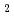In generally so far, analysis of quasi-elastic neutron scattering spectra needs some mathematical models in its process, and hence the obtained result is a model dependent. In this context, we are trying to develop new model-free analysis method which we call as mode distribution analysis. In this method, we supposed that all modes can be described as combinations of the relaxations based on the exponential law. In the result of the analysis, we can obtain an intensity distribution for HWHM of Loretzian. This function can show the number of modes and distributions of the relaxation times in the modes and we call it as mode distribution function (MDF). In this new approach, we can obtain the MDF by using the maximum entropy method (MEM). We will also report a first application of our method to HO at RT. The measurement was carried out on AMATERAS spectrometer installed at J-PARC. In the result, we discover a motion of water molecule, which has not reported by earlier studies.